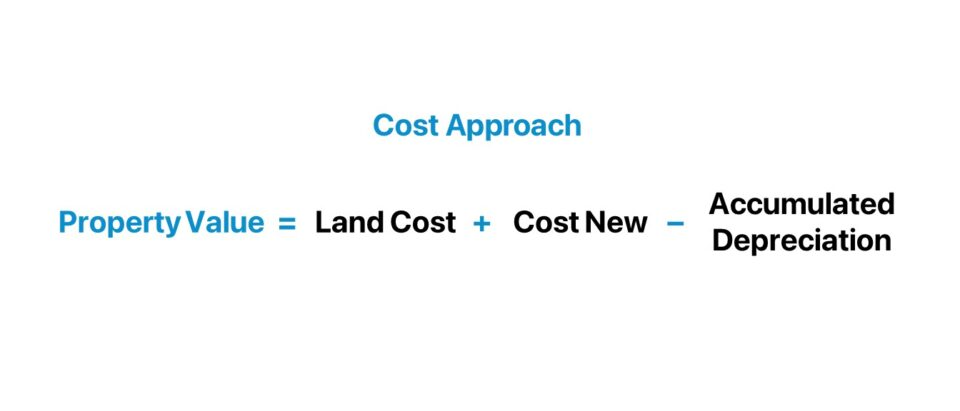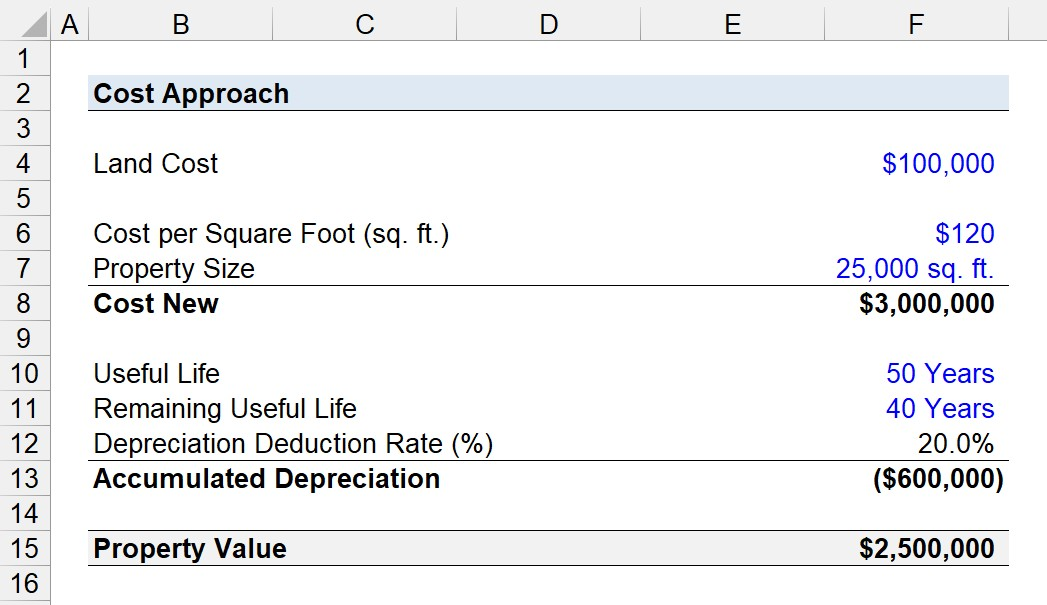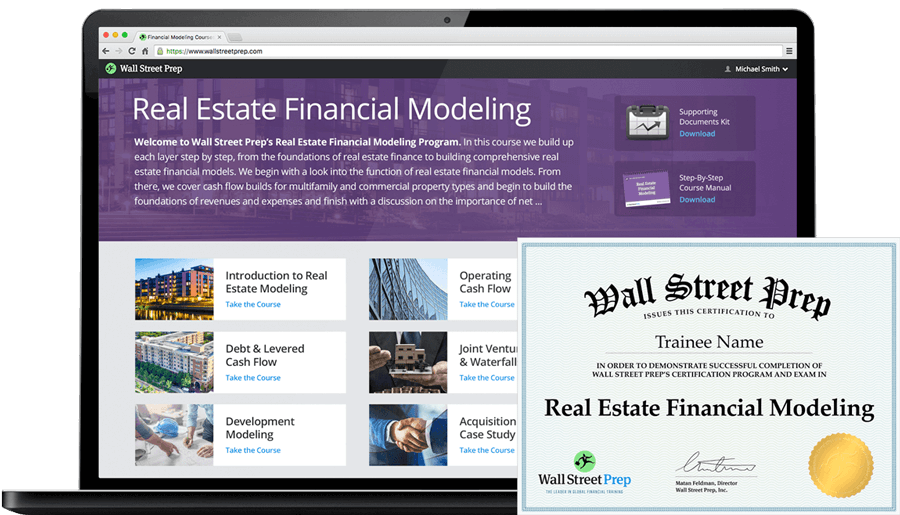Welcome to Wall Street Prep! Use code at checkout for 15% off.# Cost Approach

Guide to Understanding the Cost Approach Appraisal in Real Estate## How Does the Cost Approach Appraisal Work?

The cost approach is among one of the three main approaches used in practice to appraise the value of real estate properties.

The two other valuation methods frequently used are the income approach and sales comparison approach. Contrary to the other approaches, the cost approach is far less reliant on the active real estate market (and any data on comparable assets).

Simply put, the cost approach estimates the value of a property based on the total cost expected to be incurred under the hypothetical pretense that the property were destroyed and needed to be reconstructed.

Therefore, the cost approach estimates the value of a property based on the value of the underlying land on which the property was constructed, the replacement (or reproduction) cost, and the accumulated depreciation of the improvements.

Briefly put, the question answered here is, “How much would it cost in total to reconstruct and re-build the property from the ground up?”

The utility of the cost approach method stands out among the three main appraisal methods for properties when there is no (or very limited) market data on comparable properties. Hence, the cost approach is often the only viable option available in such instances.

The premise of the cost approach, or “replacement cost,” is the principle of substitution, which states that no rational investor should pay more for a property than the cost of constructing an equivalent substitute.

Estimating the property cost in this case is essentially determining the total spending required to rebuild the property from the ground up, which is then compared to the current asking price:

• Replacement Cost < Asking Price → Current Pricing is Potentially Reasonable
• Replacement Cost > Asking Price → Current Pricing is NOT Reasonable## How to Calculate Property Value with the Cost Approach?

The steps to appraise the value of a property using the cost approach method are as follows.

1. Estimate Land Value → Estimate the value of the land by analyzing recent sale data on comparable, vacant land parcels. Based on the characteristics of the subject project, discretionary adjustments most likely must be applied to factor in the differences.
2. Estimate Replacement Cost of Improvements → Estimate the cost to reconstruct the property and improvements, inclusive of direct and indirect costs such as materials, labor, construction, contractor fees, permits, administrative fees, financing costs, professional fees, insurance, etc. Most often, either the square foot method or unit-in-place method is used here.
3. Estimate Accumulated Depreciation → Estimate the depreciation expense, which pertains to the losses in property value from age, wear and tear, deterioration in building components, functional obsolescence, and external obsolescence.
4. Calculate Sum of Land Value and Depreciated Improvement Cost → The implied property value under the cost approach method equals the estimated land value, net of the accumulated depreciation cost tied to the improvements.

## Cost Approach Formula

The formula for the cost approach method to perform a real estate appraisal is as follows.

Property Value = Land Value + (Cost New Accumulated Depreciation)

Where:

• Land Value → The estimated value of the land by itself, based on recent comparable sales data.
• Cost New → The cost expected to be incurred if the property was reconstructed to a nearly identical state as of the present date.
• Accumulated Depreciation → The total depreciation to date from all sources, i.e. physical depreciation, functional obsolescence, and external obsolescence.

To briefly elaborate on the “Cost New” input in the formula, there are two distinct definitions to take note of:

1. Replacement Cost New → The replacement cost is the current amount it would cost to rebuild a property using current standards for construction material, layout, and design.
2. Reproduction Cost New → In contrast, the reproduction cost is the cost to rebuild the property using identical materials, design, and standards that were in place on the date of the initial construction.

While the relative difference between the replacement and reproduction cost is marginal for modern, recently built properties, the difference in the cost can be material for historical, outdated properties, or unique real estate assets.

Further, there are three forms of depreciation recognized in real estate appraisals:

1. Physical Deterioration → The tangible losses in property value from the “wear and tear” starting from the initial date on which the construction was completed.
2. Functional Obsolescence → The losses in the market value of a property due to factors such as subjective consumer preferences, technological innovations, or unfavorable shifts in market standards.
3. Economic Obsolescence → The losses in property value caused by external factors that pertain to the property’s location, such as the local economy and environment.

## Cost Approach Calculator

We’ll now move to a modeling exercise, which you can access by filling out the form below.#### Excel TemplateSubmitting...

## Cost Approach Calculation Example

Suppose a real estate appraiser is tasked with estimating the value of a residential property using the cost approach.

Based on the data collected on land sales of comparable size and in a nearby location, the appraiser estimates the land on which the residential property stands to be currently worth around \$100k.

• Land Cost = \$100,000

Using the comparative unit method, a form of analysis to determine the cost of construction on a square footage basis (and with consideration toward the construction and material type, as well as quality), the appraiser prices the cost at \$120 per sq. ft.

• Cost per Square Foot (sq. ft.) = \$120

If we assume the building is \$25k sq. ft. in total, the cost new is \$3 million.

• Property Size = 25,000 sq. ft.
• Cost New = \$120 × 25,000 sq. ft. = \$3 million

The economic useful life of the improvements is estimated to be 50 years with 40 years remaining.

The age-life method of depreciation, assuming straight-line depreciation and a salvage value of zero, implies that the improvements must be depreciated by 20.0%.

• Depreciation Deduction (% Aged) = 1 – (40 Years ÷ 50 Years) = 20.0%

After applying the depreciation deduction of 20.0% to the cost new of \$3 million, the accumulated depreciation amounts to \$600k.

• Accumulated Depreciation = 20.0% × \$3 million = \$600,000

In closing, the final step is to add the land cost to the depreciation-adjusted cost new to arrive at \$2.5 million for the value of the residential property per the cost approach.

• Property Value = \$100k + \$3 million – \$600k = \$2.5 million20+ Hours of Online Video Training

### Master Real Estate Financial Modeling

This program breaks down everything you need to build and interpret real estate finance models. Used at the world's leading real estate private equity firms and academic institutions.

Inline Feedbacks